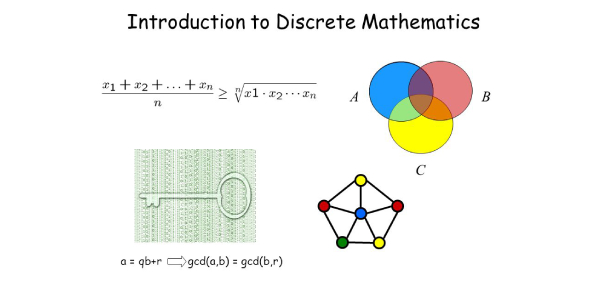# The Ultimate Discrete Math MCQ Quiz

19 Questions | Total Attempts: 43Settings• 1.
If the eccentricity of the hyperbola x2 - y2sec2θ = 5 is √3 times the eccentricity of the ellipse x2sec2θ + y2 = 25, then tan2θ is
• A.

2

• B.

1

• C.

3

• D.

1/2

• 2.
If the ellipse x2/4 + y2 = 1 meets the ellipse x2 + y2/a = 1 in two distinct points and a = b2 - 10b + 25, then the the value of b is cannot be:
• A.

1

• B.

2

• C.

3

• D.

All of the above

• 3.
The number of 4 letter words (with or without meaning) that can be formed from the letters of the word EXAMINATION is
• 4.
Two women and some men participated in a chess tournament in which every participant played two games with each of the other participants. If the number of games that the men played between themselves exceeds the number of games that the men played with the women by 66, then the number of men who participated in the tournament lies in the interval:
• A.

(14,17)

• B.

[8,9]

• C.

(11,13]

• D.

[10,12)

• 5.
A committee of 11 members is to be formed from 8 men and 5 women. If m is the number of ways the committee is formed with at least 6 men and n is the number of ways the committee is formed with at least 3 women, then:
• A.

N = m - 8

• B.

M + n = 68

• C.

M = n = 78

• D.

M = n = 68

• 6.
The number of 4 digit numbers strictly greater than 4321 that can be formed using the digits 0,1,2,3,4,5 (repetition of the digits is allowed) is:
• A.

360

• B.

288

• C.

310

• D.

306

• 7.
If the area of the triangle whose one vertex is at the vertex of the parabola y2 + 4(x - a2) and the other two vertices are the points of intersection of the parabola and y-axis, is 250 sq units, then a value of 'a' is:
• A.

5√5

• B.

5

• C.

(10)2/3

• D.

5(21/3)

• 8.
From 6 different novels and 3 different dictionaries, 4 novels and 1 dictionary are to be selected and arranged in a row on a shelf so that the dictionary is always in the middle. Then the number of such arrangements is
• A.

Less than 500

• B.

At least 500 but less than 750

• C.

At least 750 but less than 1000

• D.

At least 1000

• 9.
If a hyperbola has length of its conjugate axis equal to 5 and distance between its foci is 13, then the eccentricity of the hyperbola is:
• A.

13/8

• B.

13/6

• C.

2

• D.

13/12

• 10.
The equation y2/(1+r) − x2/(1−r) = 1
• A.

Represents a hyperbola of eccentricity equal to 2/(r+1)1/2  if r ∈ (0,1)

• B.

Represents a hyperbola of eccentricity equal to (1-r)1/2 / (1+r)1/2  if r ∈ (0,1)

• C.

Represents a ellipse of eccentricity equal to (2)1/2 / (r+1)1/2 if r>1

• D.

Represents a ellipse of eccentricity equal to (r+1)1/2 / (2)1/2  if r>1

• 11.
A man X has 7 friends, 4 of them are ladies and 3 are men. His wife Y also has 7 friends, 3 of them are ladies and 4 are men. Assume X and Y have no common friends. Then the total number of ways in which X and Y together can throw a party inviting 3 ladies and 3 men, so that 3 friends of each of X and Y are in the party, is
• A.

469

• B.

484

• C.

485

• D.

468

• 12.
An 8 digit number divisible by 9 is to be formed by using the digits from 0 to 9 without repeating the digits. In how many ways, can this be done?
• A.

72 x 7!

• B.

18 x 7!

• C.

40 x 7!

• D.

36 x 7!

• 13.
Find the rank of the word PATNA from the end if all its permutations are arranged in all possible ways as in a dictionary.
• A.

42

• B.

19

• C.

18

• D.

43

• 14.
The length of the chord of the parabola x2 = 4y having equation x - √2 y + 4√2 = 0 is:
• A.

8√2

• B.

2√11

• C.

6√3

• D.

3√11

• 15.
If the vertices of the hyperbola be at (−2,0) and (2,0) and one of the foci be at (-3,0) then which one of the following points does not lie on the hyperbola?
• A.

(-6, 2√10)

• B.

(2√6, 5)

• C.

(4,√15)

• D.

(6,5√2)

• 16.
The number of common tangents to circles x2+y2-4x-6y-12=0 and x2+y2+6x+18y+26=0 is:
• A.

1

• B.

2

• C.

3

• D.

4

• 17.
The circle passing through (1,-2) and touching the x-axis at (3,0) also passes through the point:
• A.

(2,-5)

• B.

(5,-2)

• C.

(-2,5)

• D.

(-5,2)

• 18.
Let T(n) be the number of all possible triangles formed by joining the vertices of an n-sided regular polygon. If T(n+1) - T(n) = 10, then the value of n is:
• A.

5

• B.

10

• C.

8

• D.

7

• 19.
In how may ways, can 6 girls and 4 boys be seated in a row so that no two boys are together ?
• A.

604800

• B.

304200

• C.

152100

• D.

1209600

Related TopicsBack to top